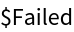Function Repository Resource:

# ParameterizePolygon

Find the parameterization of a curve that linearly connects a list of points

Contributed by: Garrett Dubofsky, Wolfram|Alpha Math Team
 ResourceFunction["ParameterizePolygon"][{p1,p2,…},t] parameterizes the polygon defined by points pi with variable t from 0 to 1. ResourceFunction["ParameterizePolygon"][{{p11,p12,…},{p21,…},…},t] parameterizes the curve defined by a collection of polygons. ResourceFunction["ParameterizePolygon"][{p1,p2,…},{t,tmin,tmax}] creates a parameterization with variable t from tmin to tmax. ResourceFunction["ParameterizePolygon"][{p1,p2,…},{t0,tmin,tmax}] evaluates the parameterization with domain tmin to tmax at t0.

## Details

The collection of points pi can be in any dimension.
Points pi can be a combination of symbolic and numeric values.
The parameterization evaluates to {} for any inputs outside the domain.
The speed of the parameterization is consistent; the Euclidean distance between pairs of points is proportional to the domain allocated to the line connecting the points.
The following options are supported:
 "ClosedCurve" True whether to connect the first and last points "ExactValues" True the precision of the output "Orientation" Automatic the order used to evaluate the list of points

## Examples

### Basic Examples (2)

Parameterize the line from {0,1} to {1,1} over the domain[0,1]:

 In:=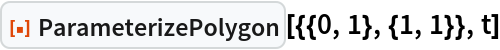Out=Parameterize the 3D polygon formed by three points:

 In:=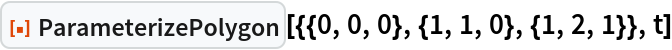Out=Parameterize the same polygon over the domain[-3,3]:

 In:=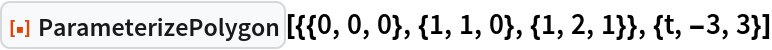Out=Evaluate the above parameterization at t=-1/2:

 In:=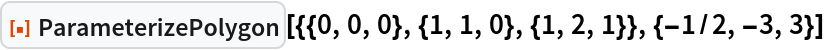Out=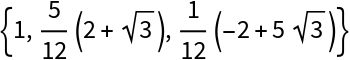### Scope (6)

Parameterize a collection of polygons:

 In:=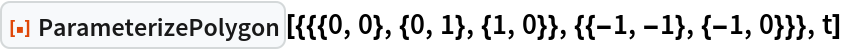Out=Find the linear parameterization between a combination of numeric and symbolic points:

 In:=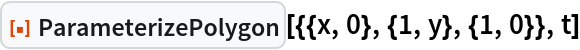Out=Parameterize a 1D curve:

 In:=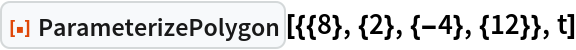Out=Parameterize curves in 4 dimensions or greater:

 In:=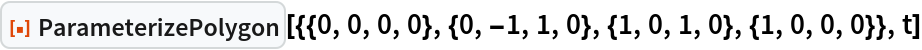Out=The list of points can be substituted with Rectangle instead:

 In:=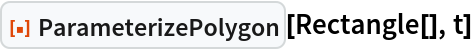Out=Substituting with Triangle is also supported:

 In:=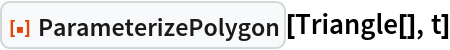Out=Besides Triangle[], the heads Line, Triangle, and Polygon are ignored:

 In:=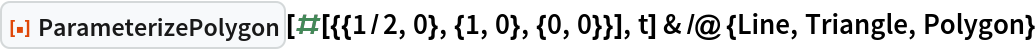Out=The resulting piecewise function can be input into ParametricPlot or ParametricPlot3D:

 In:=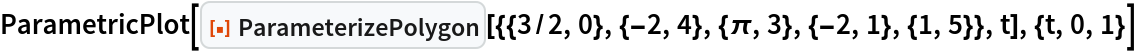Out=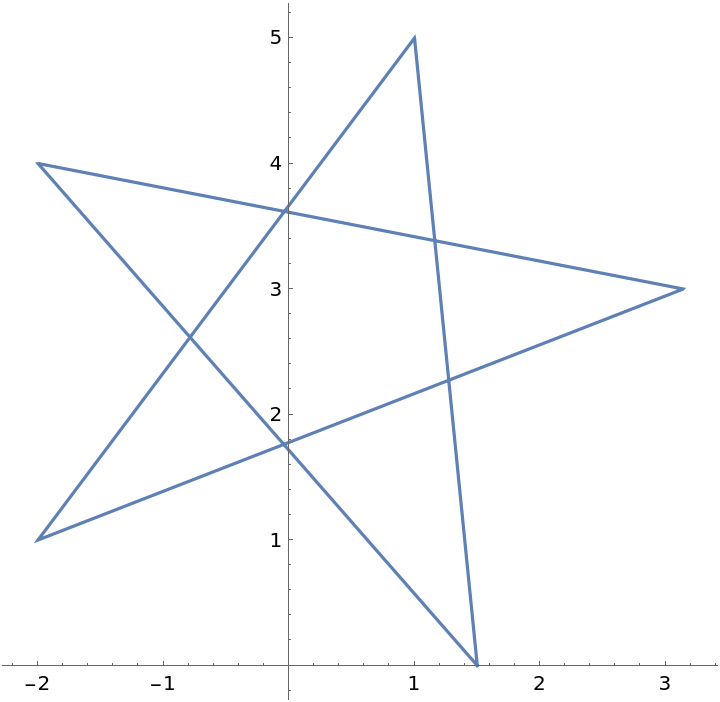In:=Out=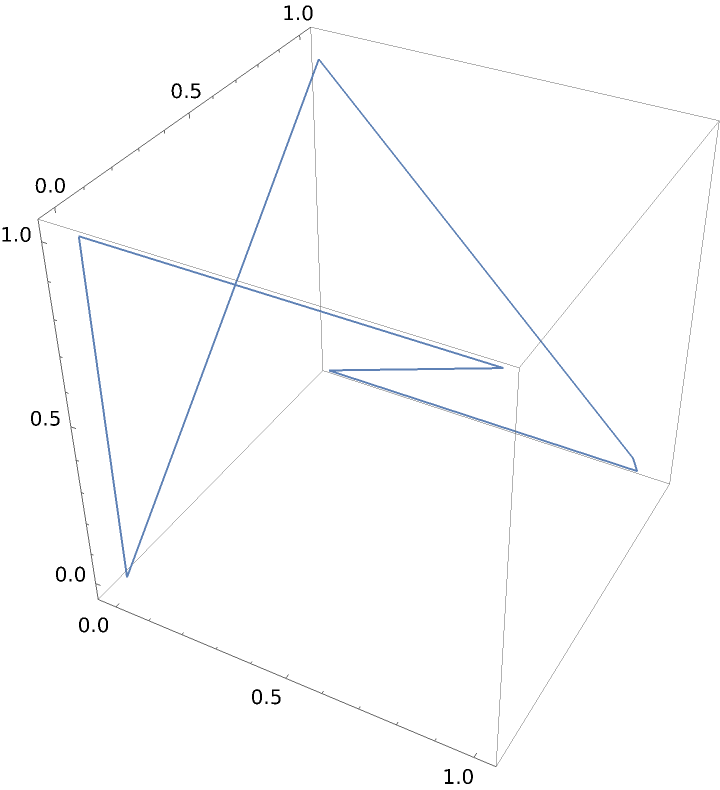Pairs of adjacent points that are the same are treated as a single point:

 In:=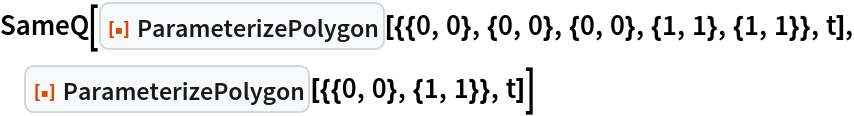Out=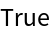### Options (3)

Determine if the list of points defines a closed curve:

 In:=Out=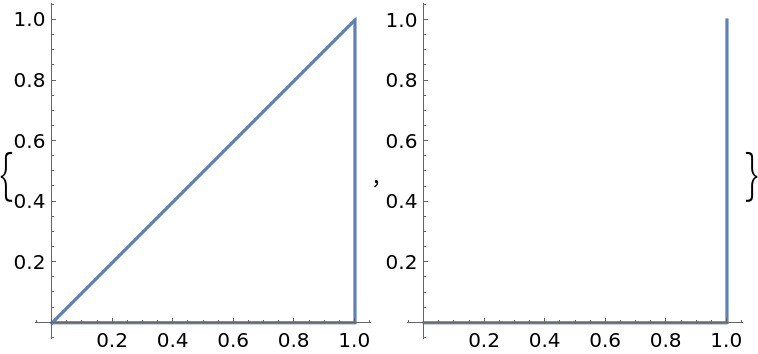Each polygon within a collection is automatically closed:

 In:=Out=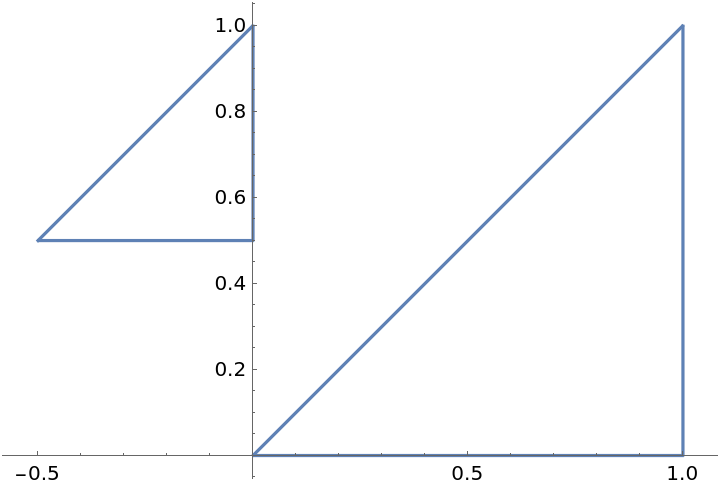Setting "ExactValues" to False causes the output to be imprecise:

 In:=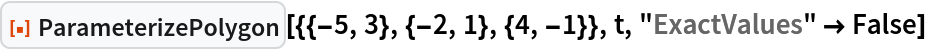Out=Determine the order of which the points are transversed using the "Orientation" option:

 In:=Out=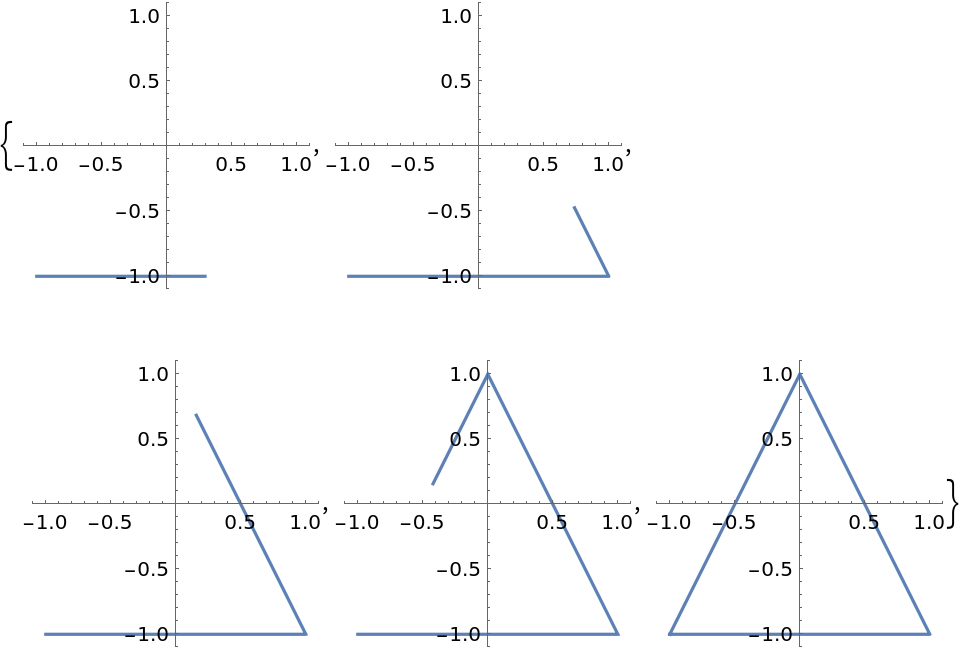In:=Out=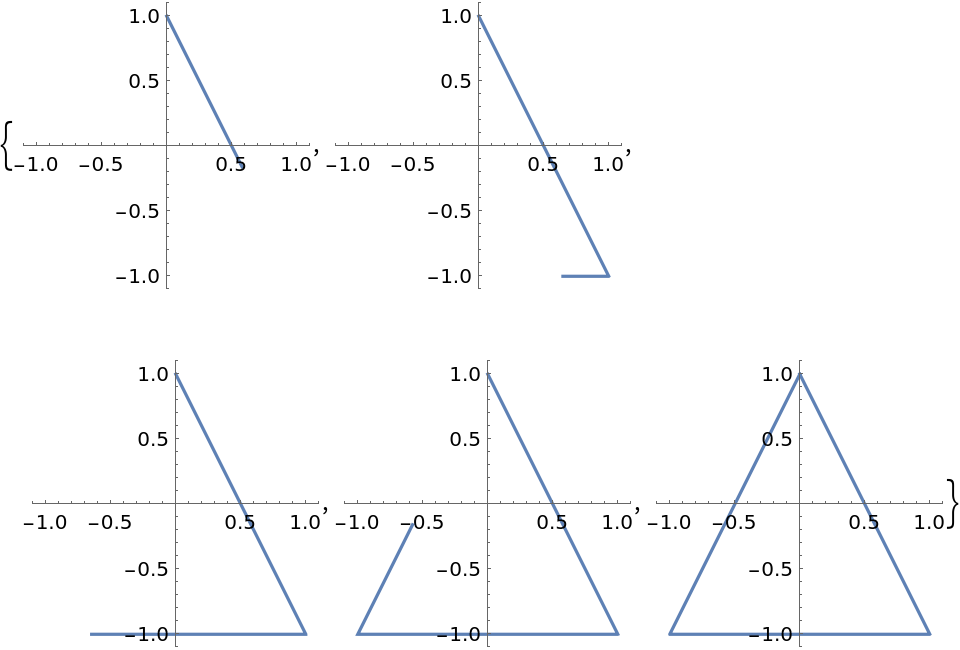Curves formed using Rectangle are transversed clockwise by default:

 In:=Out=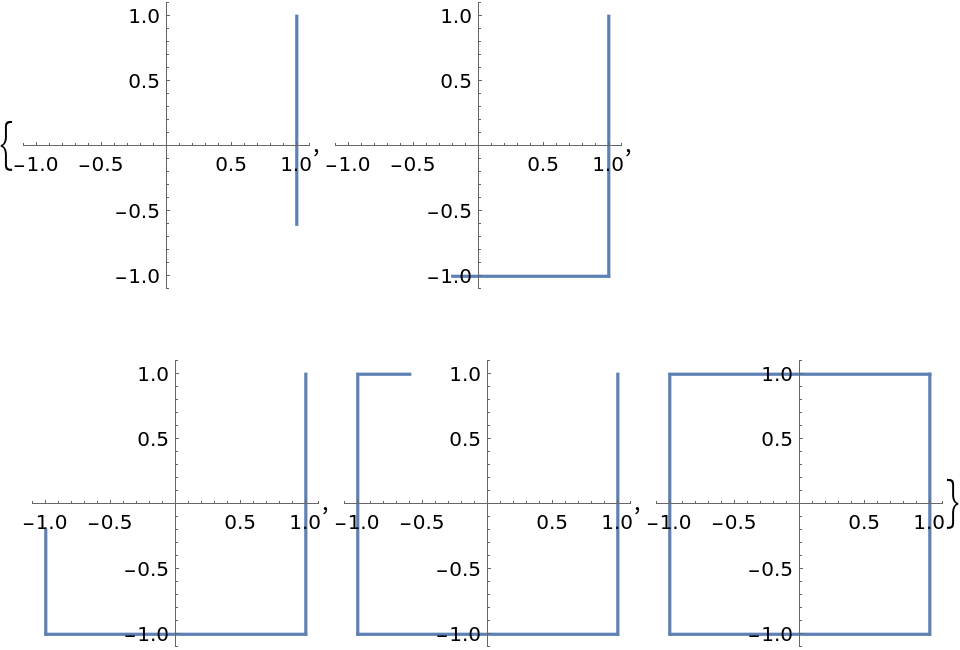The curve formed from Triangle[] is transversed counterclockwise by default:

 In:=Out=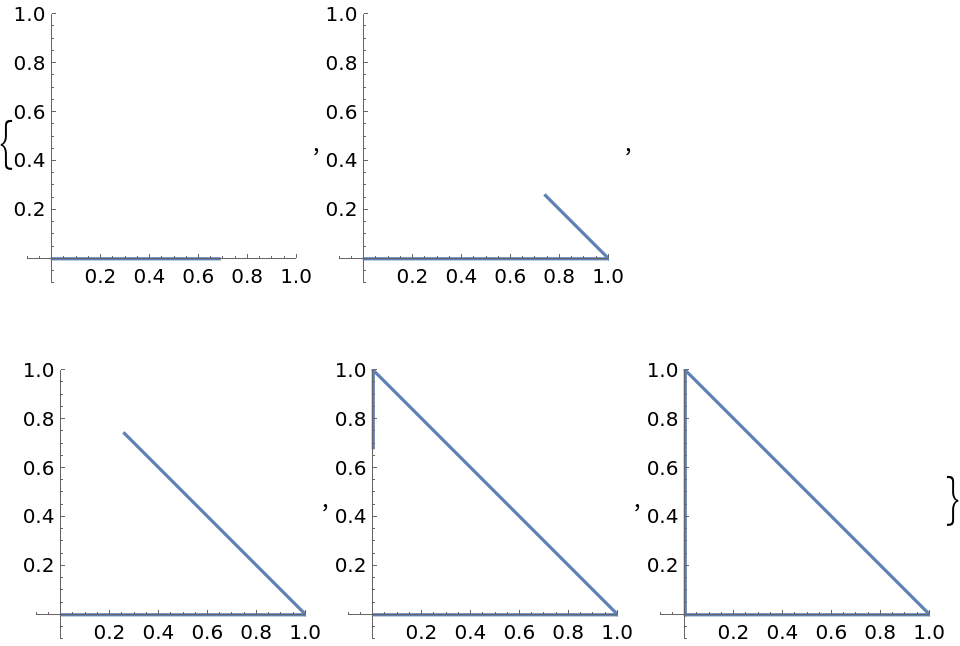### Applications (5)

Use the parameterization to analyze a function with 2D domain along a given curve:

 In:=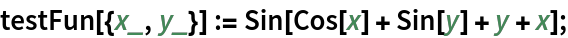In:=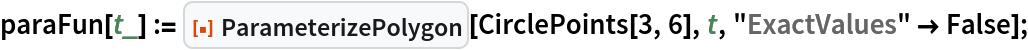Flatten out the 3D curve to see its output along the parameterization:

 In:=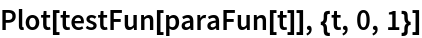Out=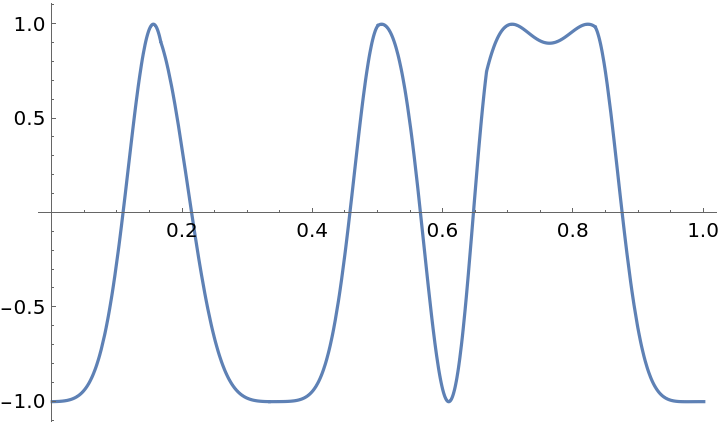Superimpose the output along the parameterization atop the 3D function curve:

 In:=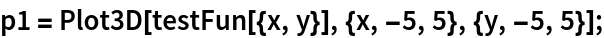In:=In:=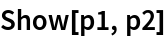Out=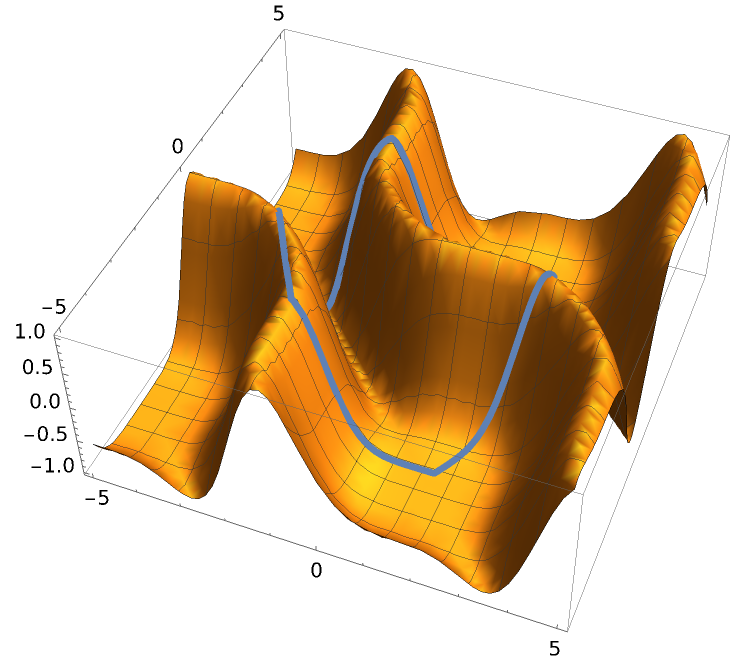Visualize the output of a function with three variables along a 3D polygon:

 In:=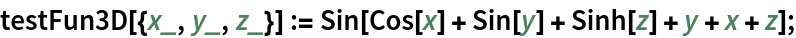In:=Plot the polygon with Hue shading corresponding to the value of the function:

 In:=Out=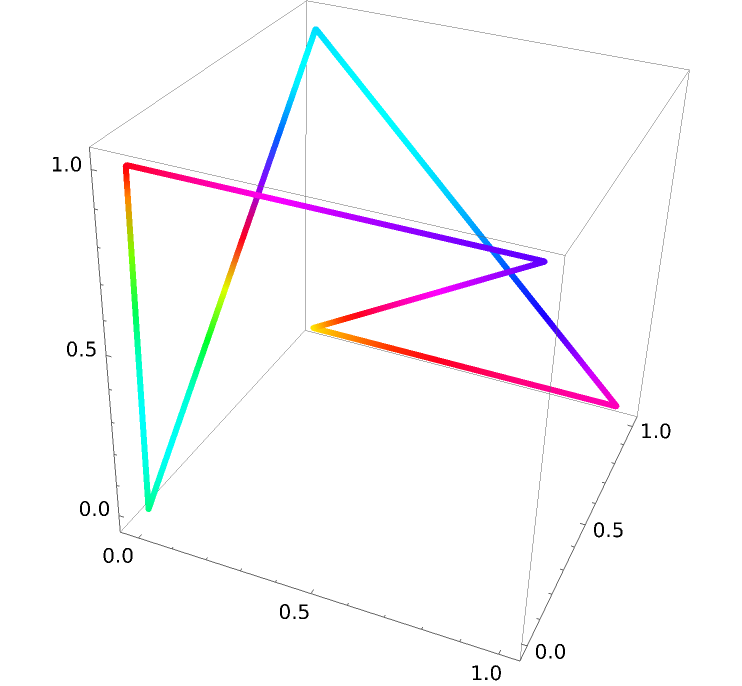Plot the same function values as a timeline of the parameterization:

 In:=Out=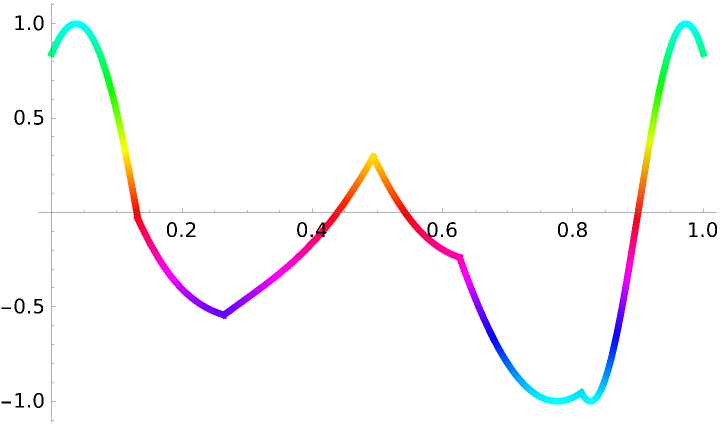This process can be generalized to find the output of a 4 variable function along a 4D curve:

 In:=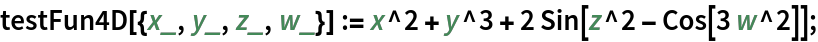In:=In:=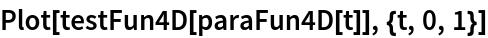Out=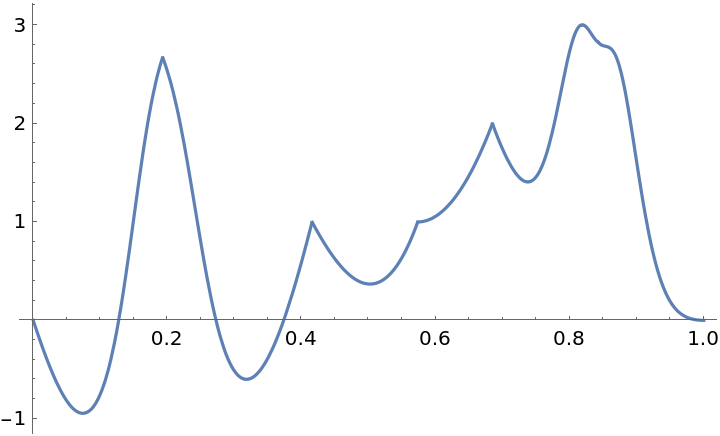Higher-dimensional polygons can be visualized using Norm along their parameterization:

 In:=Out=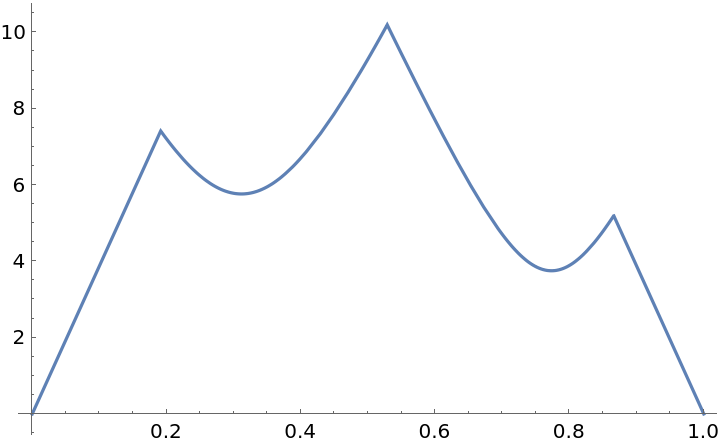Linear functions can be generated using the plots of 1D polygons:

 In:=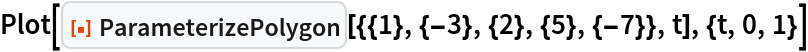Out=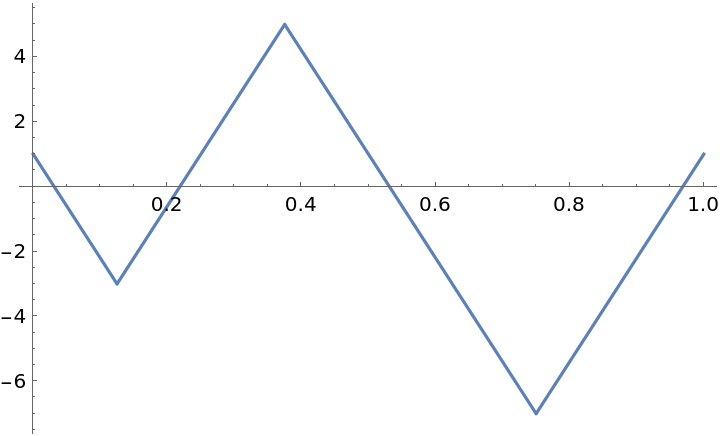Regular polygons can be parameterized:

 In:=Out=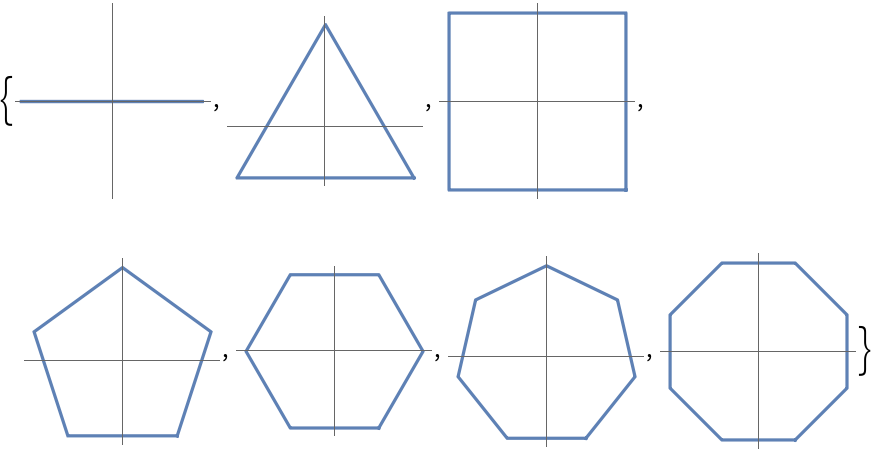Other named curves can also be parameterized:

 In:=Out=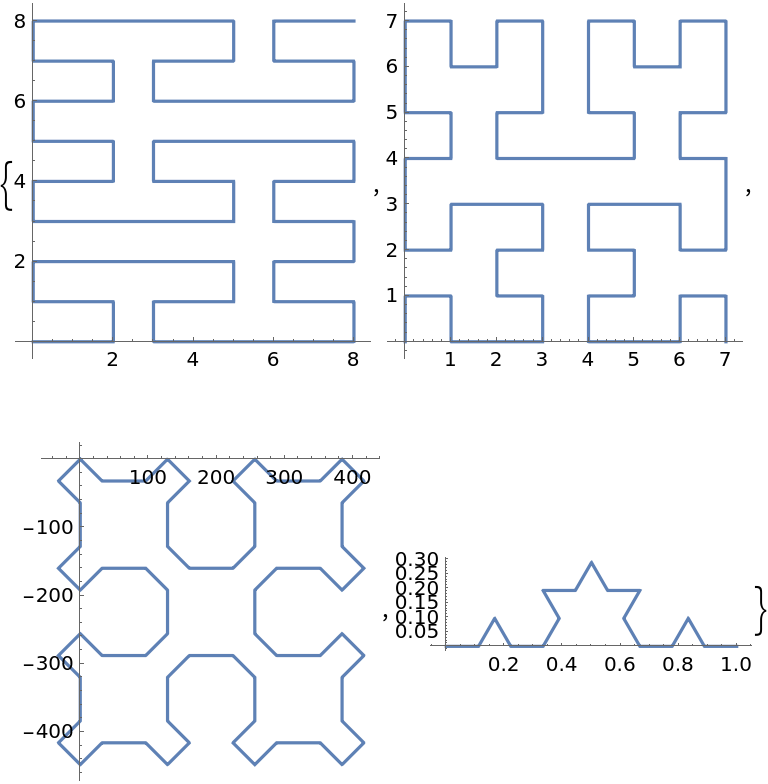### Properties and Relations (1)

The resource function ColorWinding uses ParameterizePolygon internally and to generate the "PolygonPlot" output:

 In:=Out=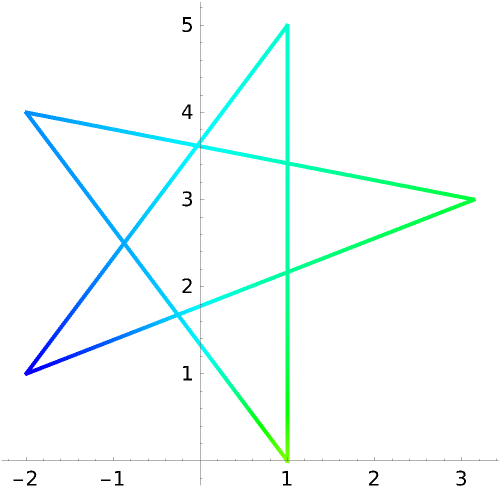### Possible Issues (4)

Plotting functions are more efficient when the parameterization has inexact values:

 In:=Out=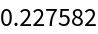In:=Out=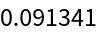Some polygons encounter a precision error that can be resolved by setting "ExactValues" to False:

 In:=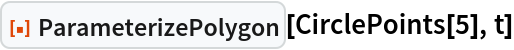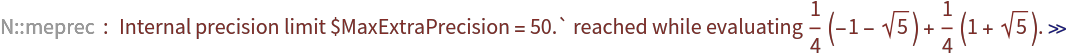Out=In:=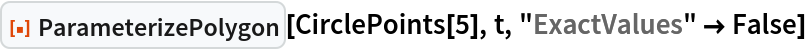Out=Sometimes ParametricPlot will include a connecting line between multiple polygons:

 In:=Out=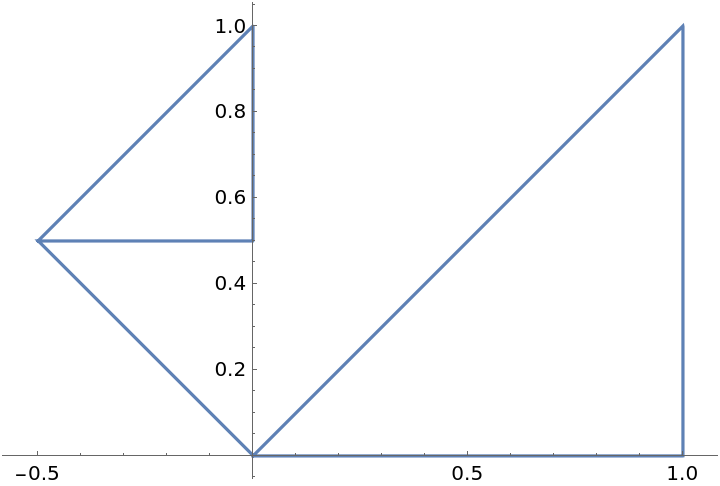Increasing the number of PlotPoints will remove extraneous points along the curve:

 In:=Out=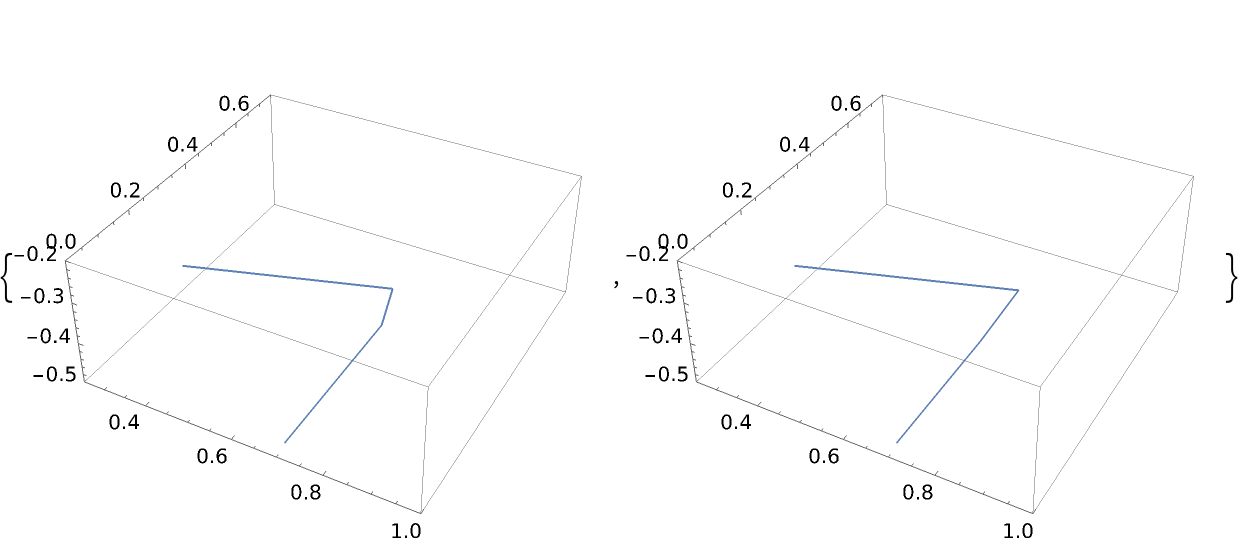### Neat Examples (3)

Plot the parameterization of a cube:

 In:=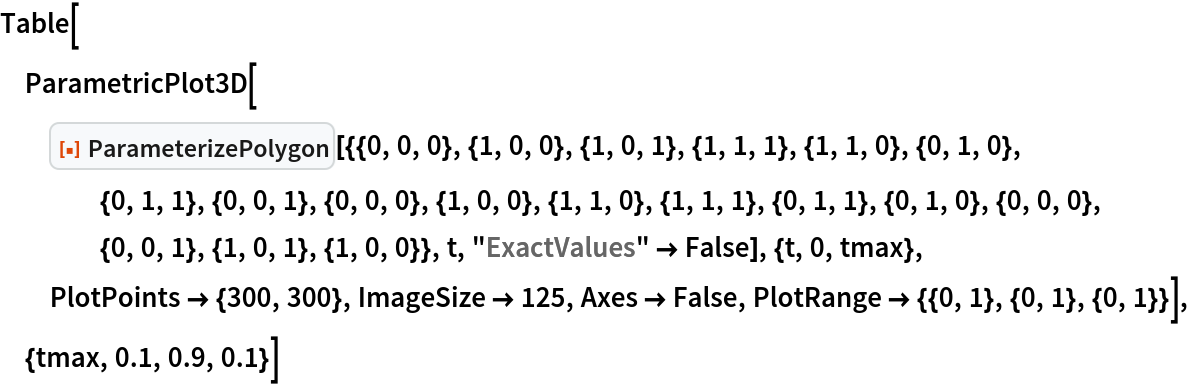Out=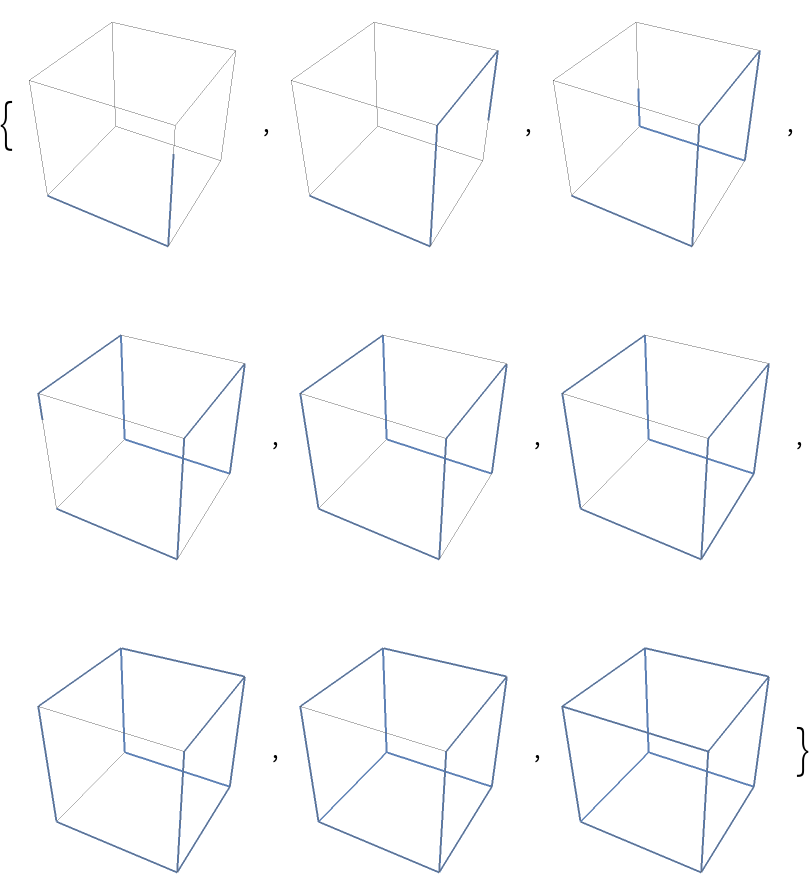Create abstract shapes using a single parameterization:

 In:=Out=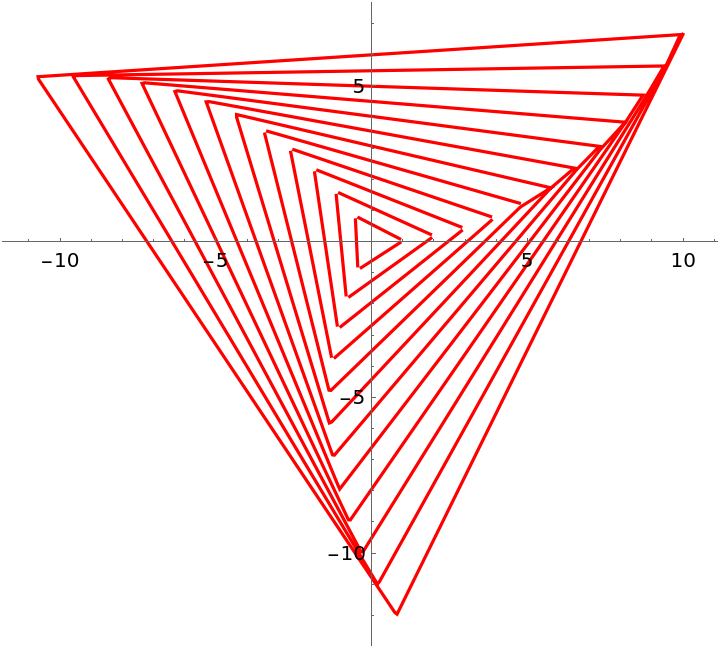Applying a function along a 2D parameterization adds a new dimension to the shape:

 In:=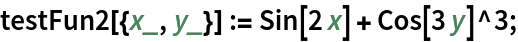In:=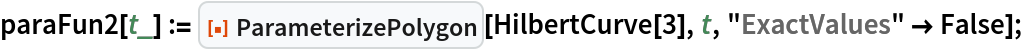In:=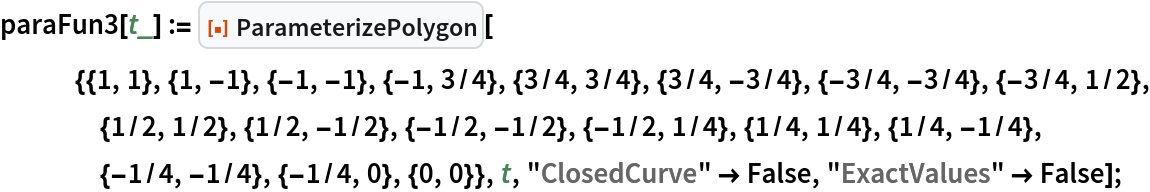In:=Out=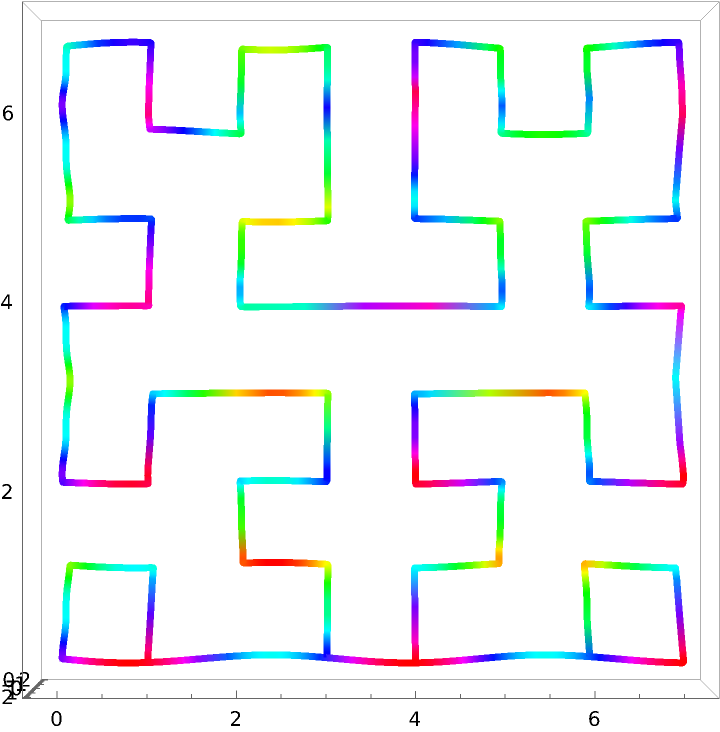In:=Out=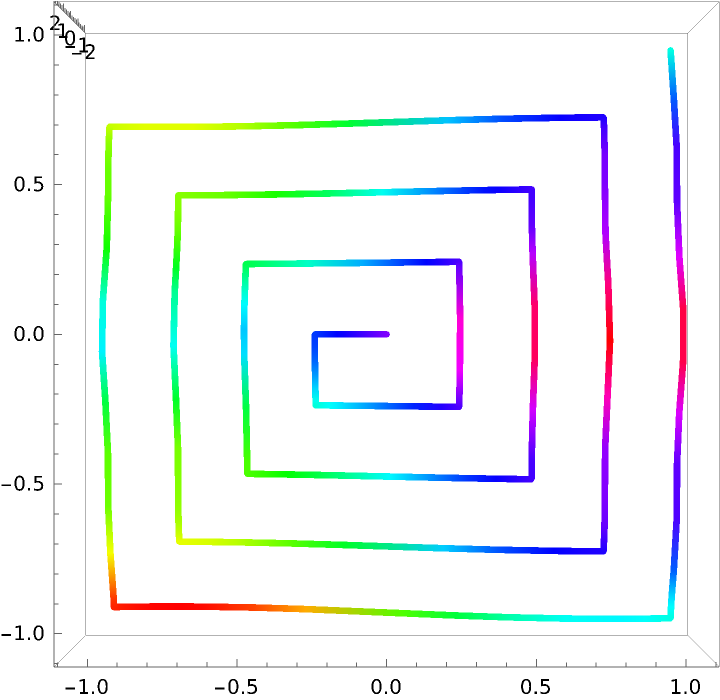Garrett Dubofsky

## Version History

• 1.0.0 – 29 August 2022

## Author Notes

The Piecewise function defaults to {} so that ParametricPlot and ParametricPlot3D do not encounter an error when evaluating the function outside the specified domain.

As seen in ParameterizePolygon[{{1},{2},{3}},t], each 1D point is surrounded by brackets to distinguish them from a higher-dimension point such as {1,2,3}.

To view the full source code for ParameterizePolygon, evaluate the following: